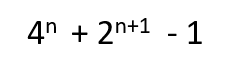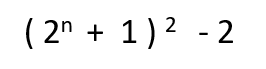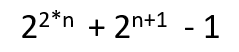Open in App
Not now

# Program to find the nth Kynea number

• Last Updated : 29 Aug, 2022

Given a positive integer n, the task is to find nth Kynea number.
Kynea number: In mathematics, a Kynea number is a positive integer of the form:where n is a positive integer.
The equivalent formula for nth Kynea number is:The first few Kynea number are:

7, 23, 79, 287, 1087, 4223, 16639, 66047, 263167, 1050623, 4198399, …..

Examples:

Input: 2
Output: 23
Putting n = 2 in formula,
= 42 + 2 2+1 – 1
= 16 + 8 -1
= 23

Method 1: A Simple Solution is to find out the nth number by putting the value of n in the formulaBelow is the implementation of the above approach:

## C++

 `// CPP code to find nth Kynea number`   `#include ` `using` `namespace` `std;`   `// Function to calculate nth kynea number` `long` `nthKyneaNumber(``int` `n)` `{`   `    ``// Calculate nth kynea number` `    ``// using formula ((2^n + 1)^2 ) -2`   `    ``// Firstly calculate 2^n + 1` `    ``n = (1 << n) + 1;`   `    ``// Now calculate (2^n + 1)^2` `    ``n = n * n;`   `    ``// Now calculate ((2^n + 1)^2 ) - 2` `    ``n = n - 2;`   `    ``// return nth Kynea number` `    ``return` `n;` `}`   `// Driver Program` `int` `main()` `{` `    ``int` `n = 8;`   `    ``// print nth kynea number` `    ``cout << nthKyneaNumber(n);`   `    ``return` `0;` `}`

## Java

 `// JAVA code to find nth Kynea number`   `class` `GFG {`   `    ``// Function to calculate nth kynea number` `    ``static` `long` `nthKyneaNumber(``int` `n)` `    ``{`   `        ``// Calculate nth kynea number` `        ``// using formula ((2^n + 1)^2 ) -2`   `        ``// Firstly calculate 2^n + 1` `        ``n = (``1` `<< n) + ``1``;`   `        ``// Now calculate (2^n + 1)^2` `        ``n = n * n;`   `        ``// Now calculate ((2^n + 1)^2 ) - 2` `        ``n = n - ``2``;`   `        ``// return nth Kynea number` `        ``return` `n;` `    ``}`   `    ``// Driver Program` `    ``public` `static` `void` `main(String[] args)` `    ``{` `        ``int` `n = ``2``;`   `        ``// print nth kynea number` `        ``System.out.println(nthKyneaNumber(n));` `    ``}` `}`

## Python

 `# Python code to find nth Kynea number`   `# Function to calculate nth kynea number ` `def` `nthKyneaNumber( n): ` `    `  `    ``# Calculate nth kynea number` `    ``# using formula ((2 ^ n + 1)^2 ) -2` `    `  `    ``# Firstly calculate 2 ^ n + 1` `    ``n ``=` `( ``1` `<< n) ``+` `1` `    `  `    ``# Now calculate (2 ^ n + 1)^2 ` `    ``n ``=` `n ``*` `n` `    `  `    ``# Now calculate ((2 ^ n + 1)^2 ) - 2` `    ``n ``=` `n``-``2` `    `  `    `  `    ``# return nth Kynea number` `    ``return` `n` `    `      `# Driver Code ` `n ``=` `2`   `# print nth kynea number` `print``(nthKyneaNumber(n))`

## C#

 `// C# code to find nth Kynea number`   `using` `System;` `class` `GFG {`   `    ``// Function to calculate nth kynea number` `    ``static` `long` `nthKyneaNumber(``int` `n)` `    ``{`   `        ``// Calculate nth kynea number` `        ``// using formula ((2^n + 1)^2 ) -2`   `        ``// Firstly calculate 2^n + 1` `        ``n = (1 << n) + 1;`   `        ``// Now calculate (2^n + 1)^2` `        ``n = n * n;`   `        ``// Now calculate ((2^n + 1)^2 ) - 2` `        ``n = n - 2;`   `        ``// return nth Kynea number` `        ``return` `n;` `    ``}`   `    ``// Driver Program` `    ``public` `static` `void` `Main()` `    ``{` `        ``int` `n = 2;`   `        ``// print nth kynea number` `        ``Console.WriteLine(nthKyneaNumber(n));` `    ``}` `}`

## PHP

 `

## Javascript

 ``

Output:

`66047`

Time Complexity: O(1), only constant operations are being used.
Auxiliary Space: O(1), as no extra space is required.

Method 2: This solution is based on the fact that every Kynea number follows a specific pattern in their binary representation. nth Kynea number can be represented in binary as a single leading one followed by exactly n-1 consecutive 0’s, followed by n+1 consecutive 1’s.

Example:

```23 is 2nd kynea number
It can be represented in binary as 10111
(Single leading one, followed by n - 1 ( i.e 2-1=1 ) consecutive 0's,
followed by n + 1 ( i.e 2 + 1 = 3 ) consecutive 1's.)```

Observing the binary pattern of Kynea number in above table, the nth Kynea number can be easily calculated using the formula:Example:

Input: n = 3
Output: 79
Using formula,
= 26 + 24 -1
= 64 + 15
= 79

Below is the implementation of the above approach

## C++

 `// CPP code to find nth Kynea number`   `#include ` `using` `namespace` `std;`   `// Function to calculate nth kynea number` `long` `nthKyneaNumber(``int` `n)` `{`   `    ``// Calculate nth kynea number` `    ``return` `((1 << (2 * n)) + (1 << (n + 1)) - 1);` `}`   `// Driver Program` `int` `main()` `{` `    ``int` `n = 2;`   `    ``// print nth kynea number` `    ``cout << nthKyneaNumber(n);`   `    ``return` `0;` `}`

## Java

 `// JAVA code to find nth Kynea number`   `class` `GFG {`   `    ``// Function to calculate nth kynea number` `    ``static` `long` `nthKyneaNumber(``int` `n)` `    ``{`   `        ``// Calculate nth kynea number` `        ``return` `((``1` `<< (``2` `* n)) + (``1` `<< (n + ``1``)) - ``1``);` `    ``}`   `    ``// Driver Program` `    ``public` `static` `void` `main(String[] args)` `    ``{` `        ``int` `n = ``2``;`   `        ``// print nth kynea number` `        ``System.out.println(nthKyneaNumber(n));` `    ``}` `}`

## Python

 `# Python code to find nth Kynea number`   `# Function to calculate nth kynea number ` `def` `nthKyneaNumber( n): `   `    ``# Calculate nth kynea number    ` `    ``return` `(( ``1` `<< (``2` `*` `n)) ``+` `( ``1` `<< (n ``+` `1``)) ``-``1` `) ` `    `    `# Driver Code ` `n ``=` `2`   `# print nth kynea number` `print``(nthKyneaNumber(n))`

## C#

 `// C# code to find nth Kynea number`   `using` `System;` `class` `GFG {`   `    ``// Function to calculate nth kynea number` `    ``static` `long` `nthKyneaNumber(``int` `n)` `    ``{`   `        ``// Calculate nth kynea number` `        ``return` `((1 << (2 * n)) + (1 << (n + 1)) - 1);` `    ``}`   `    ``// Driver Program` `    ``public` `static` `void` `Main()` `    ``{` `        ``int` `n = 2;`   `        ``// print nth kynea number` `        ``Console.WriteLine(nthKyneaNumber(n));` `    ``}` `}`

## PHP

 ``

## Javascript

 ``

Output:

`23`

Time Complexity: O(1), only constant operations are being used.
Auxiliary Space: O(1), as no extra space is required.

My Personal Notes arrow_drop_up
Related Articles